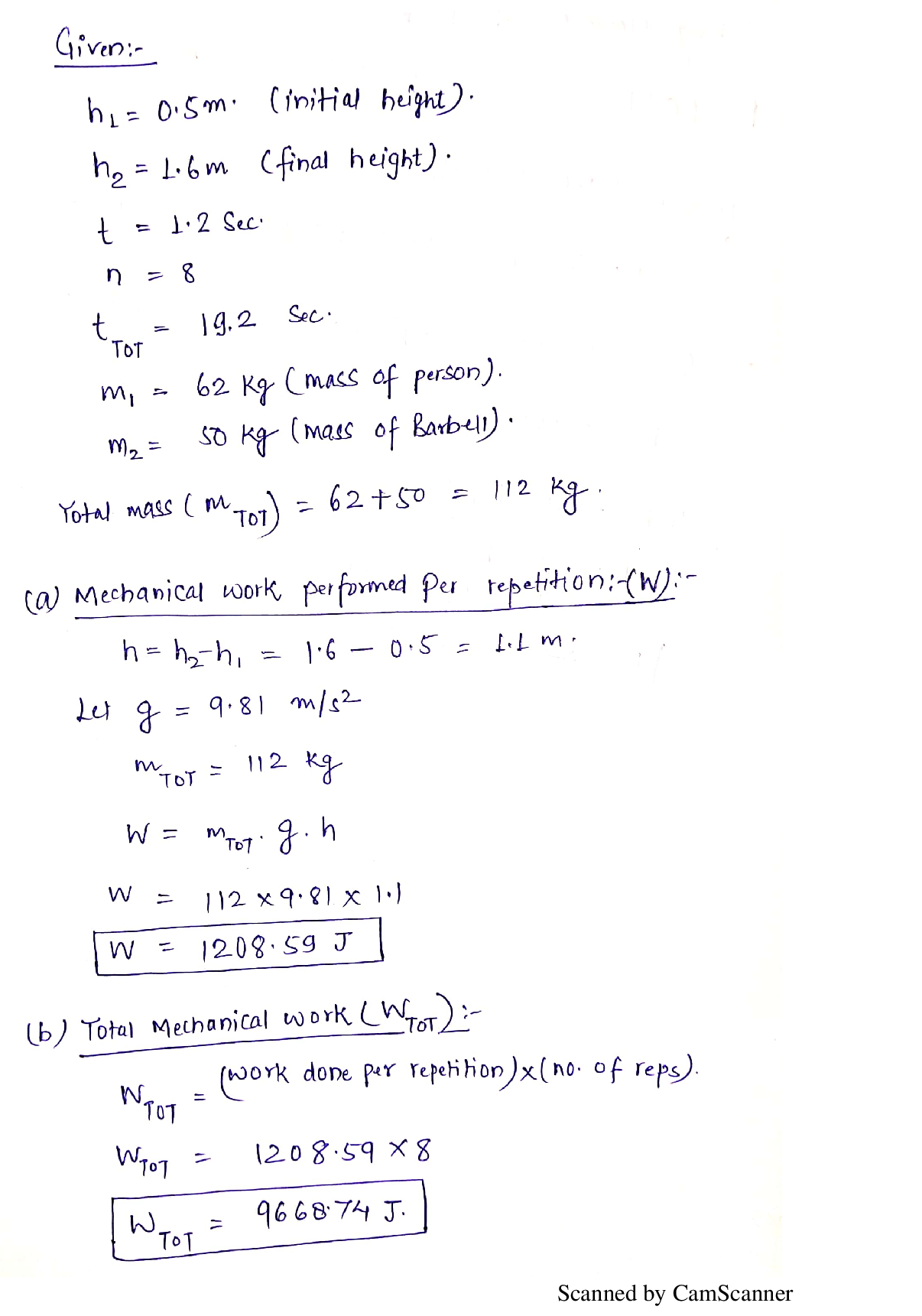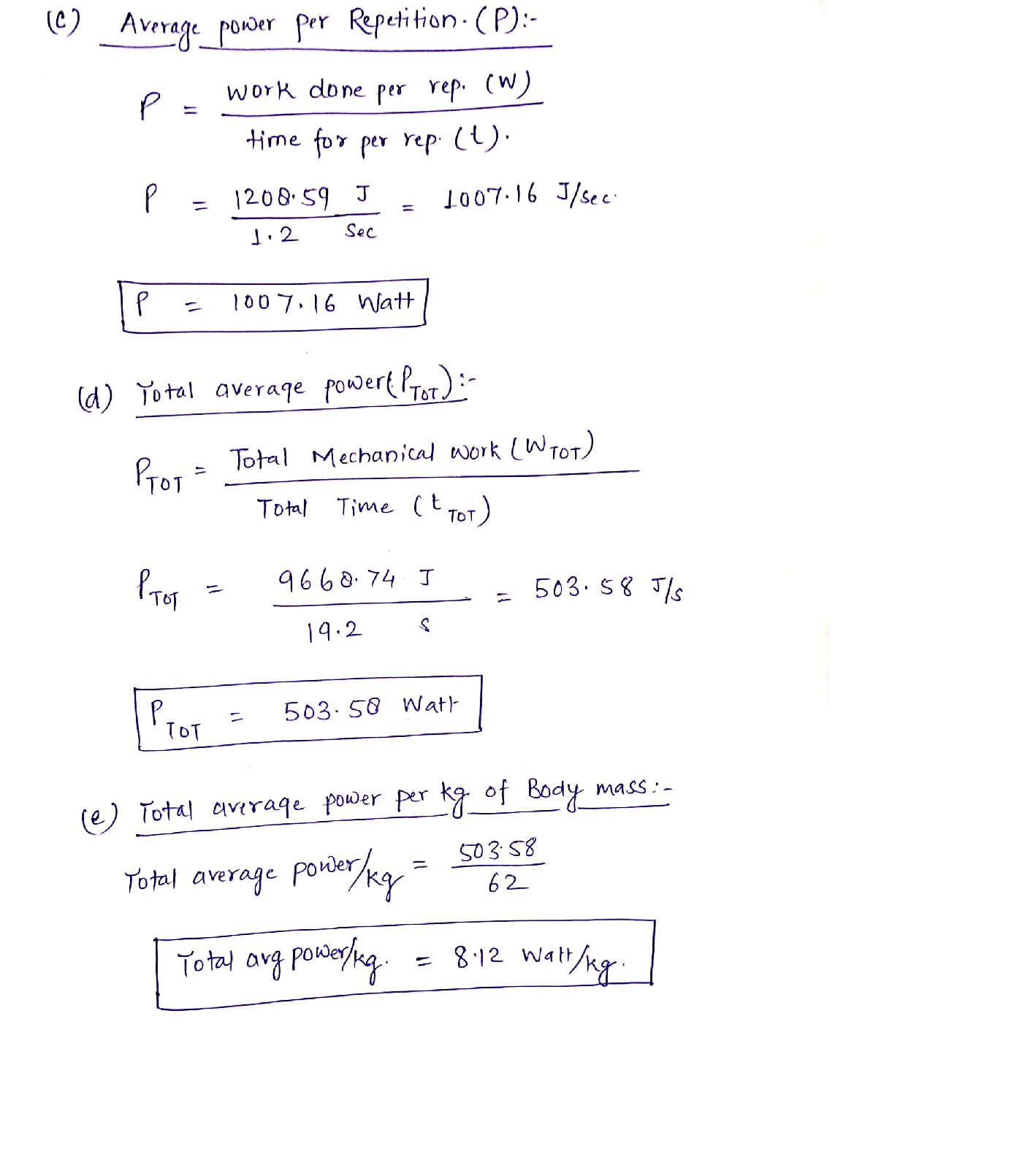Question

Biomechanics

6. A person is performing a set of squats. The time for the upward movement and the downward movement is the same, assume that when squatting down no work is performed.

m=62kg (mass of the person)

h2=1.6 m (final height of total center of mass)

h1= 0.5m (initial height of total center of mass)

trợr-19.2s (total time)

n=8 (number of repetitions)

t=1.2 s (time to go from h1 to h2)

total average power

mechanical work performed per repetition

m2=50kg (mass of the barbell)

total mechanical work

average power per repetition

total average power per kg of body massVerified### Question 40905Biomechanics

6. A person is performing a set of squats. The time for the upward movement and the downward movement is the same, assume that when squatting down no work is performed.
m=62kg (mass of the person)
h2=1.6 m (final height of total center of mass)
h1= 0.5m (initial height of total center of mass)
trợr-19.2s (total time)
n=8 (number of repetitions)
t=1.2 s (time to go from h1 to h2)
total average power
mechanical work performed per repetition
m2=50kg (mass of the barbell)
total mechanical work
average power per repetition
total average power per kg of body mass

### Submit query